﻿ Digital Circuits and Systems - Circuits i Sistemes Digitals (CSD) - EETAC - UPCBachelor's Degree in Telecommunications Systems and in Network Engineering## Circuits based on logic gates

1. Analysis especifications

Deduce the truth table of the Circuit_P represented in Fig. 1 using method III (Boole's algebra).

Check results analysing again the circuit using method IV (VHDL synthesis and simulation).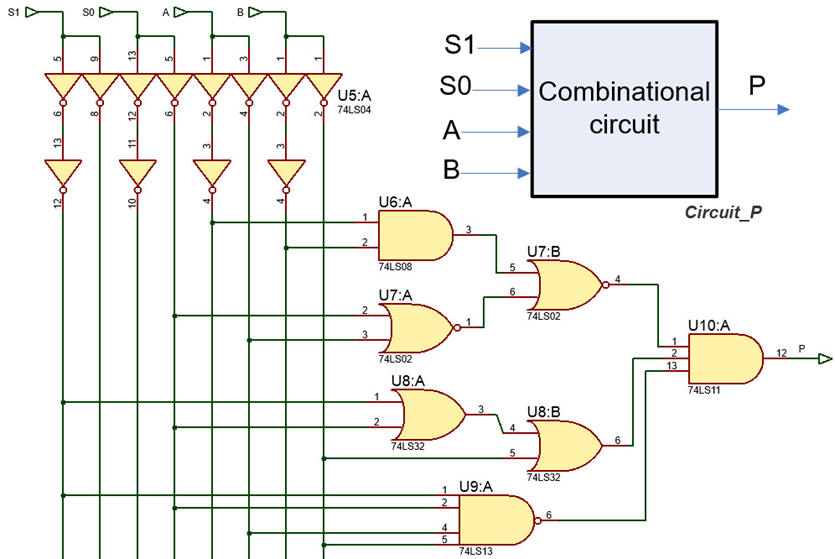Fig. 1. Circuit_P based on logic gates.

2. Planning the analysis

Fig. 2 shows the concepts and steps involved in method III and method IV for analysing the given circuit.Fig. 2. Several methods for analysing a simple circuit based on gates.

1. Deduce the circuit's equation and apply Boole's algebra to get firstly the SoP or the PoS and secondly, the sum of minterns or product of maxterms, which are conceptually the truth table itself.

2. Start a VHDL project, synthesise the circuit for a target chip, and run a simulation testbench considering all input combinations to observe and write down the truth table from the waveform diagram.

3. Developing the analysis project

Apply Boolean algebra to deduce the truth table and minterms or maxterms. Circuit_P notes.The truth table as a product of maxterms: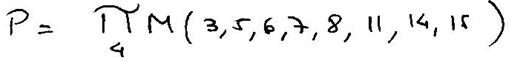4. Analysis validation

Method IV: translate the circuit's equation into VHDL and synthesise the circuit starting a single-file ispLEVER Classic or Diamond / Quartus Prime / ISE or Vivado project. Choose a from vendors. This is the Circuit_P.vhd equation as the VHDL source file for this project.

C:\CSD\P1\Circuit_P\VHDL\(files)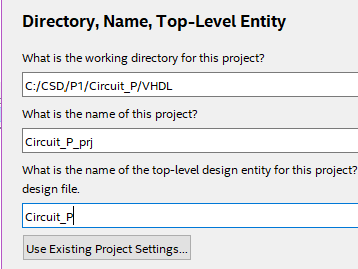Fig. 3. Project folder and names.

Inspect RTL and technology views.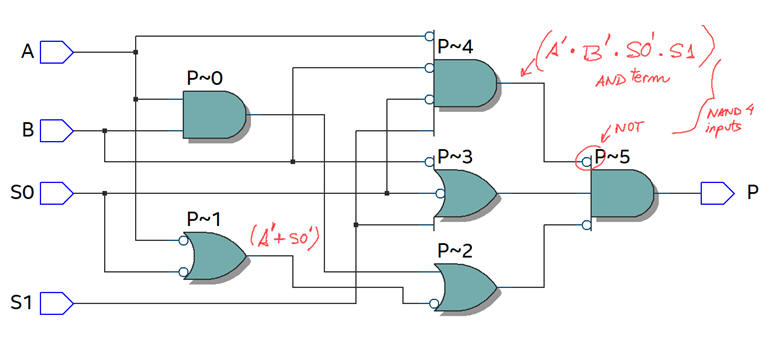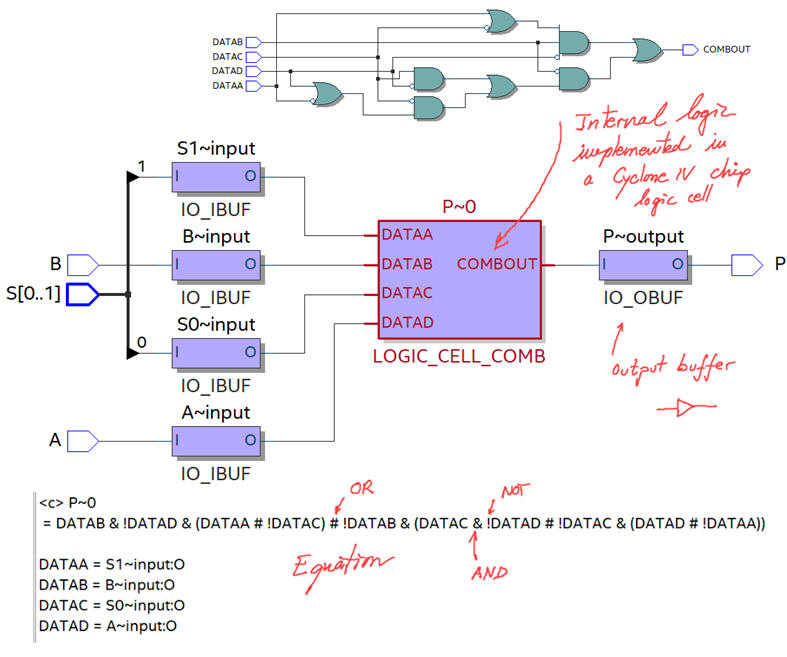Fig. 4. RTL and technology views (target chip Cyclone IV FPGA EP4CE115F29C7).

Use a testbench process Circuit_P_tb.vhd and a VHDL simulation tool (ActiveHDL, ModelSim ISim, etc.) to write down the truth table (in this file pay attention only to the stimulus process activity and the constant Min_Pulse).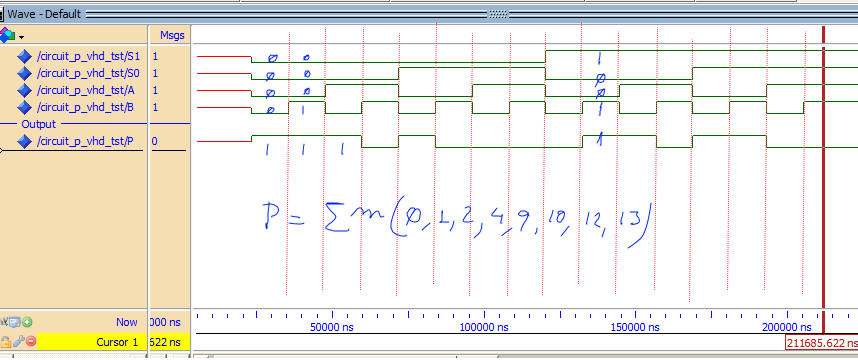Fig. 5. Waveform results to obtain the truth table.

Another alternative for testing the truth table is writing Circuit_P equation in WolframAlpha (Method II).

Another alternative for testing the truth table is capturing the circuit in Proteus Circuit_P.pdsprj and running all the combinations (Method I).

5. Analysis report

Write the project report using handwritten text and scanning printed pictures and diagrams when necessary. All the pictures must be discussed using annotations and comments.